×

Search anything:

# Counting subtrees where nodes sum to a specific value

#### Problems on Binary Tree Algorithms Data Structures tree data structureWe will learn about counting the subtrees in a binary tree whose nodes sum to a given value. We will be trying different methods to solve our problem We will solve it in O(N) time complexity and O(H) space complexity where N is number of nodes and H is the height of the tree.

## Problem: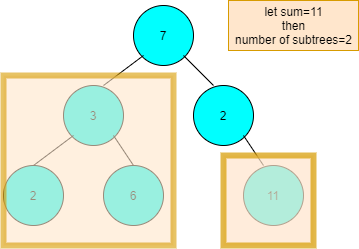Let's say we need to find count of subtrees whose nodes sum to 11.In the example subtree,the whole left subtree's nodes sum up to 11 and the leaf itself is 11.So the total number of subtrees whose nodes added up to 11 were 2.We will be seeing its algorithm,pseudocode and finally the code.
Lets look at sum basic terms of binary trees before solving this problem

### DFS and BFS:

Also called level order traversal,where the traversal takes place level wise.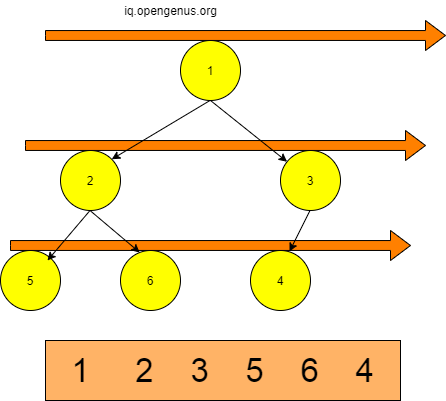#### Depth first order traversal(D.F.S):

Consists of three types of traversals:

• Inorder Traversal
It is the normal or the standard method of traversal, where the sequence of traversal is leftnode,root,rightnode .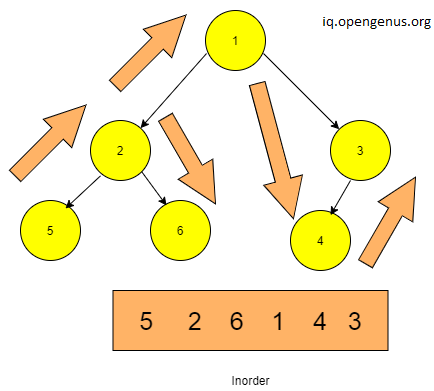• Preorder Traversal
It is the method of traversal, where the sequence of traversal is root,leftnode,rightnode .The figure given below shows the exact way it traverses.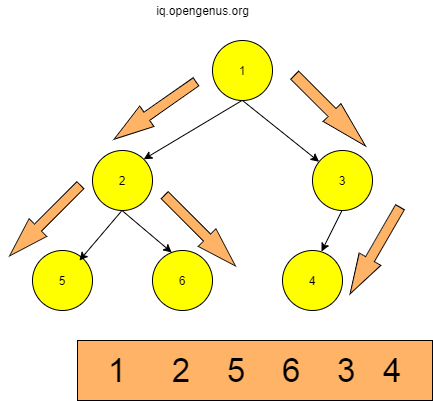First the root is traversed then the left parts and then the right subtrees.Within subtrees ,same type of traversal takes place in order of root-left-right.
• Postorder Traversal
It is the method of traversal, where the sequence of traversal is leftnode,rightnode,root .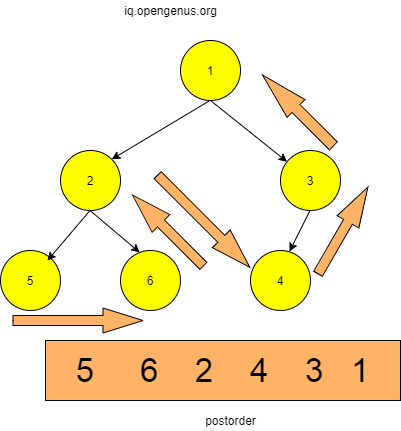### Brute Force approach:

The first approach that comes to mind when we see this question is to go to the root and check if the sum of root+leftnode+rightnode is equal to sum, else move to the next right or left node and repeat the same.Also check if entire tree's sum is equal to the given value.This would have the time complexity of O(n^2) as you check for each and every node.
So let us examine few methods through which we can count subtrees whose sum would be equal to say,x.

### 1.BFS method:

In BFS or level wise traversal , the idea is to go through each node in level wise fashion and find the subtrees of each node whose sum equals the given value and count all such subtrees.

#### Algorithm:

X=Sum to search for.
SUM=Subtree Sum(left+right+root).

1. Traverse each node by level order traversal.
2. Calculate sum of all nodes i.e. from node till the null
3. Check if SUM=X
4. If yes,Increment the count.
5. Repeat for all other nodes traversed level wise.

#### PseudoCode:

``````INT subtreeSum(ROOT){
CHECK:( ROOT=NULL)
RETURN 0
RETURN ROOT[VALUE]+subtreeSum(ROOT->LEFT)+subtreeSum(ROOT->RIGHT)
}
INT checkSubtreeSumX(ROOT,LEVEL,X,REFERENCE(count))
{
CHECK(ROOT==NULL): RETURN 0
CHECK: (LEVEL=1 && subtreeSum(ROOT)=X)
count++;

if(LEVEL>1)
{
checkSubtreeSumX(ROOT->RIGHT,LEVEL-1,X,count);
checkSubtreeSumX(ROOT->RIGHT,LEVEL-1,X,count);
}

return ROOT[VALUE];
}
INT countSubtreescheckroot(ROOT,X)
{   count=0;
h = HEIGHT(ROOT)
int i;
LOOP(i:1 to h )
checkSubtreeSumX(ROOT, i,X,count);\
RETURN count
}

``````

#### Code:

``````#include<iostream>
#include<climits>
using namespace std;
//Binary tree structure

struct node
{
int data;
struct node* left;
struct node* right;
};
//for inserting a new node
node *newNode(int data){
node *temp=new node;
temp->data=data;
temp->right=NULL;
temp->left=NULL;
return (temp);
}
//for finding the height of the tree
int height(node* root)
{
if (node == NULL)
return 0;
else
{

int lheight = height(node->left);
int rheight = height(node->right);

if (lheight > rheight)
return(lheight + 1);
else return(rheight + 1);
}
}
//for finding sum of all nodes of given subtree.
int subtreeSum(node* root)
{
if (root == NULL)
return 0;
return (root->data + subtreeSum(root->left) + subtreeSum(root->right));

}
//for checking the sum and counting subtrees whose sum=x.
int checkSubtreeSumX(node* root, int level,int x,int& count)
{
if(root==NULL)
return 0;

if(level==1 && subtreeSum(root)==x)
count++;

if(level>1)
{
checkSubtreeSumX(root->left,level-1,x,count);
checkSubtreeSumX(root->right,level-1,x,count);
}

return root->data;
}

//Executes for each level and returns total count of subtrees whose sum==x
int countSubtreescheckroot(node* root, int x)
{   int count=0;
int h = height(root);
int i;
for (i = 1; i <= h; i++)
checkSubtreeSumX(root, i,x,count);

return count;
}

int main() {
// input of sum to check for
int x;
cout<<"Enter the sum to check for"<<endl;
cin>>x;
//sample input of the binary tree
struct node* root=newNode(1);
root->left=newNode(3);
root->right=newNode(4);
root->left->right=newNode(6);
root->left->left=newNode(2);
root->right->right=newNode(11);
root->right->left=newNode(1);
root->right->right->left=newNode(11);
root->right->right->right=newNode(1);
cout<<"Number of subtrees with specific sum :"<<" "<<countSubtreescheckroot(root, x)<<endl;

return 0;
}

``````

#### Output:

``````Enter the sum to check for
1
Number of subtrees with specific sum : 2
``````

#### Output Explanation:

LEVEL-1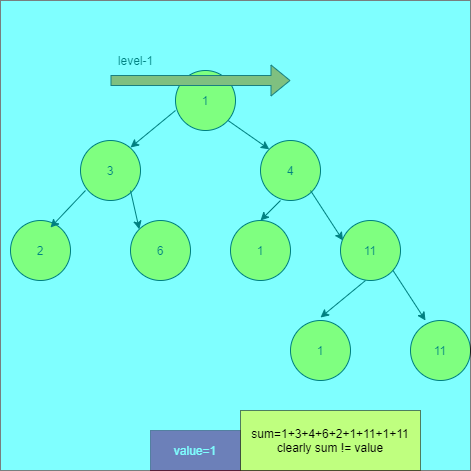Therefore count=0 now.
LEVEL-2
Left node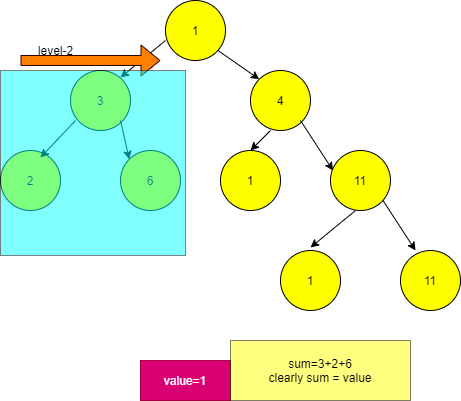The count=0 as sum=11 and sum!=value.
Right Node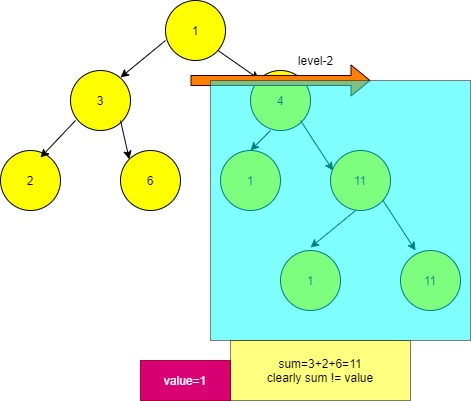Similarly it will happen for all the values whose nodes' sum is not equal to 1.
LEVEL-3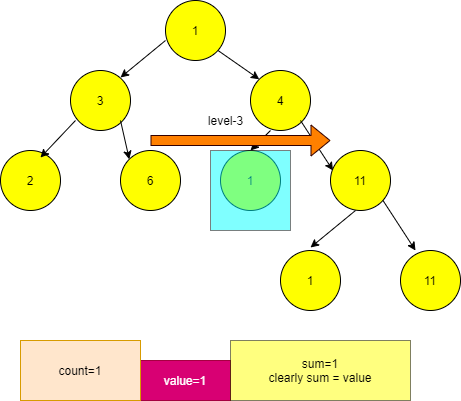As the leaf[value]=1 therefore it being equal to value , the count=1
count=1
LEVEL-4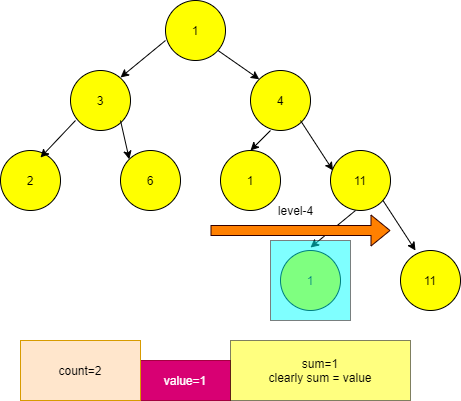Now , count=2.So later no subtrees or leaves are left , so it returns the count as 2.

#### Time and space complexity:

Time Complexity - `O(n^2)`
As the recursive call is being called for n-i times inside for loop which runs for n times , the time complexity is O(n^2) where n is number of nodes.
Space Complexity - `0(h)` where h is the height of the tree.
BFS method is better than brute force but not the best one to use considering its time complexity.We can do better by using DFS methods.

### 2.Iterative method:

We will be now using post order traversal iteratively, to find the subtrees having the total nodes sum equal to the value specified.
We will be using two stacks to traverse postorderly and then use the stack having elements in postorder fashion to find the count of the subtrees whose sum of all its nodes is equal to the given value,X.

#### Algorithm:

X=Specified value
count=Subtrees count

1. Store all nodes in a stack in postorder fashion.
2. Select the top and check if its left and right nodes exists.
3. If yes then check if the node's value is equal to X.
If the value=X then increment the count.
4. If No then:
Store the left,right nodes in a stack by calling postorder function and derive the sum by adding all the top elements and popping them up.Check if their sum=X.If yes then increment the count.
5. Pop the top and continue same steps with the next node until the stack is empty.

#### Pseudocode:

``````// stack of node* datatype.
(node*)stack postorder(ROOT){
two stacks : s1,s2
UNTIL s1 is EMPTY:
ROOT=s1.top
s1.pop
if ROOT->LEFT NOT NULL:
s1.PUSH(ROOT->LEFT)
if ROOT->RIGHT NOT NULL:
s1.PUSH(ROOT->RIGHT)
s2.PUSH(ROOT)
RETURN s2
}
INT countSubtreesum(ROOT,X,stack<node*> s){
UNTIL s is EMPTY:
if s.top->left and s.top->right are NULL
check s.top(value)=x
if yes:count++;
else
store values of each node postorderly in subs.
UNTIL subs is EMPTY:
Add all the elements to sum
if sum=X: count++
}
RETURN count
``````

#### Code:

``````#include<iostream>
#include<stack>

using namespace std;
//Binary tree structure

struct node
{
int data;
struct node* left;
struct node* right;
};
//for inserting a new node
node *newNode(int data){
node *temp=new node;
temp->data=data;
temp->right=NULL;
temp->left=NULL;
return (temp);
}
// traverses in postorder fashion but iteratively.
stack<node*> postorderTraversal(node* root) {

stack<node*> s1,s2;
if(root==NULL)
{
s2.push(NULL);
return s2;
}
s1.push(root);
while(!s1.empty()){
root=s1.top();
s1.pop();
if(root->left!=NULL)
s1.push(root->left);
if(root->right!=NULL)
s1.push(root->right);

s2.push(root);
}

return s2;
}
//Function to count all possible subtrees with sum x.
int countSubtreesum(node* root,int x,stack<node*> s){
int count=0;
while(!s.empty()){
node* root=s.top();
s.pop();
if(root->left==NULL && root->right==NULL)
{
if(root->data==x)
count++;
}
else{
stack<node*> subs=postorderTraversal(root);
int sum=0;
while(!subs.empty()){
sum=sum+subs.top()->data;
subs.pop();
}
if(sum==x)
count++;
}

}
return count;
}

int main() {
// input of sum to check for
int x;
cout<<"Enter the sum to check for"<<endl;
cin>>x;
//sample input of the binary tree
struct node* root=newNode(1);
root->left=newNode(3);
root->right=newNode(4);
root->left->right=newNode(6);
root->left->left=newNode(2);
root->right->right=newNode(11);
root->right->left=newNode(1);
root->right->right->left=newNode(11);
root->right->right->right=newNode(1);
cout<<"Number of subtrees with specific sum :"<<" "<<countSubtreesum(root, x,postorderTraversal(root))<<endl;

return 0;
}

``````

#### Output:

``````Enter the sum to check for
1
Number of subtrees with specific sum : 2
``````

#### Output Explanation:

We will see how postorder trversal is being done iteratively using two stacks: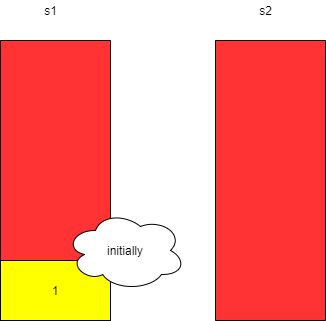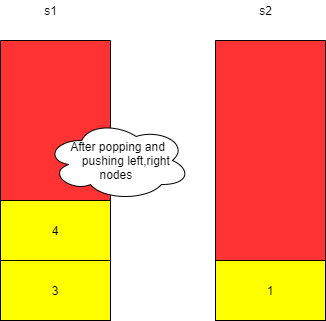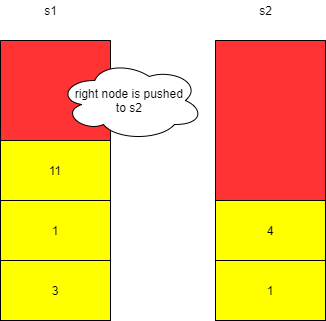Similar arrangements happen until the s1 is empty.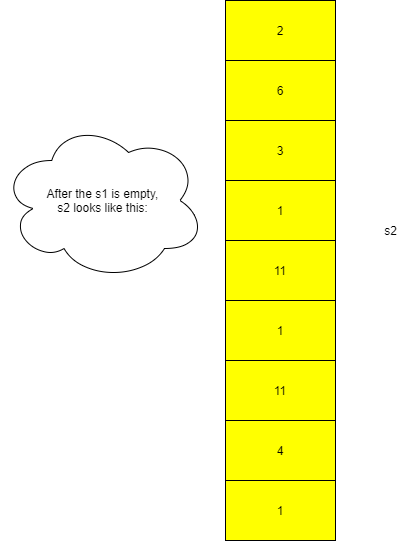The process to count the subtrees whose sum=x here x=1:
So whenever the node present in the stack points to NULL and has no right and left children,i.e if they are leaves, we just need to check if its value is equal to 1.
If yes then we increment the count.
What if the node is not NULL and points to left or right or both?
We store all the nodes of that node in a stack in a postorder way.Later we will be adding all the nodes values to the sum and checking if its equal to 1.
The tree is: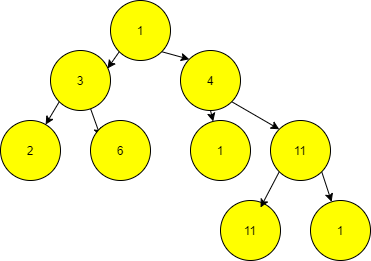So currently , the stack is :
`2 6 3 1 11 1 11 4 1`
We will take 2,store it in root and pop it, and we can see that it is a leaf and as 2!=1 ,
we will take 6, as 6!=1, same goes for it.
But 3 is not leaf so we will call postorder function and store 2,6 and 3 in subs stack.

``````sum=2
pop(2)
sum=2+6
pop(6)
sum=8+3=11
pop(3)
``````

As stack is empty we come out of loop
sum=11 and sum!=1
So no increment of count.
This will continue till stack is empty and later the count is returned.
As there are two 1s which are leafs ,so count=2.

### Time and Space Complexity:

Due to stacks being used it occupies extra space and worst time complexity is `O(n^2)`.We can counter these problems using recursive approach.

### 3.Recursive method:

#### Algorithm

We will be now using post order traversal recursively, to find the subtrees having the total nodes sum equal to the value specified.

1. If ROOT:=NULL then return 0
2. Traverse through the left subtre.
3. Traverse through the right subtree.
4. Check for LEFT[VALUE]+RIGHT[VALUE]+ROOT[VALUE]:=target sum.
5. If yes then increment the count.
6. Then return the sum of subtrees.
7. Check whether the main root and the left and right subtree sum is equal to target sum.
8. If yes then increment count.
9. Return the count of subtrees with sum equal to target sum.

#### Pseudocode

``````INT helper(ROOT,TSUM,(REFERENCE)COUNT){
CHECK:( ROOT=NULL)
RETURN 0
LEFTSUBTREE :=helper(ROOT->LEFT,TSUM,COUNT)
RIGHTSUBTREE :=helper(ROOT->RIGHT,TSUM,COUNT)
CHECK TSUM=LEFTSUBTREE+RIGHTSUBTREE+ROOT[VALUE]
IF YES: COUNT:=COUNT+1
RETURN SUM
}
INT countSubtrees(ROOT,TSUM){
COUNT:=0
CHECK:( ROOT=NULL)
RETURN 0
LEFTSUBTREE :=helper(ROOT->LEFT,TSUM,COUNT)
RIGHTSUBTREE :=helper(ROOT->RIGHT,TSUM,COUNT)
CHECK TSUM=LEFTSUBTREE+RIGHTSUBTREE+ROOT[VALUE]
IF YES: COUNT:=COUNT+1
RETURN COUNT
}
``````

#### Code

``````#include<iostream>
using namespace std;
//Binary tree structure
struct node
{
int data;
struct node* left;
struct node* right;
};
//for inserting a new node
node *newNode(int data){
node *temp=new node;
temp->data=data;
temp->right=NULL;
temp->left=NULL;
return (temp);
}
//helper function
//Function to return sum of subtrees and count subtrees with sum=x
int countSubtreesWithSumX(node* root, int x ,int& count)
{ if(root==NULL)
return 0;
int ls= countSubtreesWithSumX(root->left,x,count);
int rs= countSubtreesWithSumX(root->right,x,count);
int sum=ls+rs+root->data;
if(sum==x)
count++;
return sum;
}
//main function
//Function to return the count of subtrees whose sum=x
int countSubtreescheckroot(node* root, int x)
{
if(root==NULL)
return 0;
int count=0;
//return sum of left and right subtrees respectively
int ls= countSubtreesWithSumX(root->left,x,count);
int rs= countSubtreesWithSumX(root->right,x,count);
//checks if the root value added to sums of left and right subtrees equals x
if(x==ls+rs+root->data)
count++;
//returns the count of subtrees with their sum=x
return count;
}
int main() {
// input of sum to check for
int x;
cout<<"Enter the sum to check for"<<endl;
cin>>x;
//sample input of the binary tree
struct node* root=newNode(7);
root->left=newNode(3);
root->right=newNode(2);
root->left->right=newNode(6);
root->left->left=newNode(2);
root->right->right=newNode(11);
cout<<"Number of subtrees with specific sum :"<<" "<<countSubtreescheckroot(root, x)<<endl;

return 0;
}
``````

#### Output

``````Enter the sum to check for
11
Number of trees with specific sum : 2
``````

#### Output Explanation

``````the tree is :  7
/   \
3     2
/ \     \
2   6     11
//when helper function for left,right subtrees  called
It deals with  3     and  2
/ \          \
2   6           11
//for left subtree
2!=11 and 6!=11
but 2+3+6= 11 so count=1 and returns sum of subtree.
//for right subtree
11==11
so count=count+1
=> count=2
returns sum = 11+2 = 13
//in main function
As leftsubtree+rightsubtree+root != sum
i.e. 13+11+7 > 11
so it returns the count = 2
hence
//in int main()
the output 2

``````

## Question

#### In helper function why is the count argument declared as "int& count" ?

Unary and operator
As count is a pointer
It follows call by value
It follows call by reference
Try executing the code without keeping & after int.It will show count=0.This is due to call by reference where the value is changed in both original and formal parameters as it copies the address of the variable.So changes are reflected in actual value also.

#### Time and Space Complexity

Time complexity of this code is O(n) where n is the number of nodes of the binary tree and the space complexity of the problem is `O(h)` where h is the height of the binary tree.

Now You will know how to count number of subtrees which sum up to a specific value. Thank you.

#### Rashmitha

Final Year, B.tech (Information Technology ),Indira Gandhi Delhi Technical University For Women

Counting subtrees where nodes sum to a specific value